Display Accessibility Tools

# 7-3 Stretching and Shrinking - Concepts and Explanations

## Corresponding

Corresponding sides or angles have the same relative position in similar figures.

### Example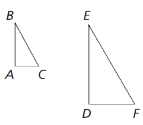• AC and DF
• AB and DE
• BC and EF

• A and D
• B and E
• C and F

## Similarity

Two figures are similar if: (1) the measures of their corresponding angles are equal and (2) the lengths of their corresponding sides increase by the same factor, called the scale factor.

### Example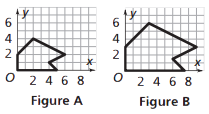The two figures at the right are similar.

The corresponding angle measures are equal.

The corresponding side lengths in Figure B are 1.5 times as long as those in Figure A.

So, the scale factor from Figure A to Figure B is 1.5. (Figure A stretches or is enlarged by a factor of 1.5, resulting in Figure B.)

We also say that the scale factor from Figure B to Figure A is 1/1.5 or 2/3. (Figure B shrinks by a factor of 2/3, resulting in figure A).

## Scale Factor

The number used to multiply the lengths of a figure to stretch or shrink it into a similar image.

A scale factor larger than 1 will enlarge a figure. A scale factor between 0 and 1 will reduce a figure.

The scale factor of two similar figures is given by a ratio that compares the corresponding sides:
length of a side on the image/ length of a side on the original.

### Example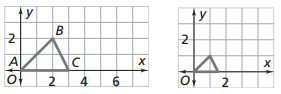If we use a scale factor of 1/2, all lengths in the images are 1/2 as long as the corresponding lengths in the original.

The base of the original triangle is 3 units.

The base of the image is 1.5 units.

The scale factor is 1.5/3 = 3/6 = 1/2.

## Area and Scale Factor

Lengths of similar figures will stretch (or shrink) by a scale factor. Areas of the figures will not change in the same way.

### Example

Applying a scale factor of 2 to a figure increases the area by a factor of 4.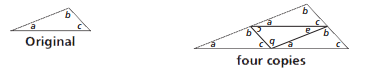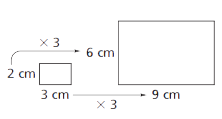Applying a scale factor of 3 to a figure, increases the area by a factor of 9. The original area is 6 cm2. The area of the image is 9 times as large (54 cm2).